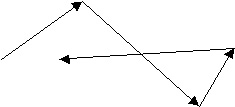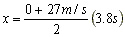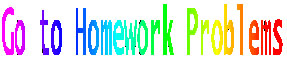Kinematics in One Dimension
 "I understand the physics; I just can't do the problems" Unknown physics student

Objects moving horizontally - fundamental terms defined

Distance & Displacement
In this opening foray into kinematics, we arbitrarily limit our consideration of motion to objects that move only in a straight line. Later, we will expand our consideration to objects that move in two dimensions.Our first consideration is the distance traveled by some object as it moved along a meandering path. In such circumstances, we are concerned only with the path length taken by the object . Distance traveled is the first example we encounter of a scalar quantity, one for which we are concerned with magnitude (size) alone and not with spatial direction. The meandering path at left represents a distance traveled that is the sum of the length of the four line segments Stated differently, if the neighborhood mail box is 20 m from your house, then mailing a letter and returning home causes you to travel a distance of 40 m.

A similar concept that is often cause for confusion is
displacement. Displacement is defined here to be the change in position from point A, the starting place, to point B at the finish. Displacement is a vector quantity. To describe any vector adequately, one must report its size and its spatial direction. The confusion comes when these terms, distance traveled and displacement, are used interchangeably, an honest error given that in some cases both quantities are numerically equivalent. In the meander above, the displacement is represented by an arrow that extends from the tail of the first vector mentioned to the head of the last. In the mail-box trip above, a person would have a distance traveled of 20 m in going to the mailbox. The magnitude of the displacement would be 20 m also. When we consider the round trip, from door step to mail box to door step, however, the matter gets more complicated. The distance traveled is now 40 m but the displacement is zero. (Go back and look at the definition of each word above.

 Remote Reading Assignments HYPERPHYSICS GLENBROOK HS M C ASCO & ASSOCIATES

 M1 Remote reading assignment 361 & 368 Mikey's sample summary

 M2 Remote reading assignment 361 368 http://www.mcanv.com/p1mot1d.html http://www.sasked.gov.sk.ca/docs/physics/u1d3phy.html

The reader should notice that appropriate (usually metric) units are installed for every quantity named. This part is critical. On exam day you will receive credit for the work that you do, except that NO UNITS = NO CREDIT Units are to be treated like numbers in an equation. This practice, often called dimensional analysis, ensures that when an equation is solved properly, the solution falls out with correct units. An important feature of dimensional analysis is that if the units are not correct for the final answer, the equation at hand probably has not been solved correctly.

It is often useful to install a convenient coordinate system when dealing with vector quantities; in this case, a convenient coordinate system might be a standard number line from an algebra course. In visiting the mail box mentioned earlier, we could (arbitrarily) arrange the coordinate system so that the front step had an address x1 = 0 and the mailbox x2 = +20 m. In this circumstance, we define displacement to be displacement = (D x) = final position - initial position = x2 - x1. (D x) = 20 m - 0 = 20 m. The displacement on the return trip would be (D x) = 0 - 20m = - 20m. Note that the algebraic sign at the front of the displacement shows the direction of the displacement, a direction that is consistent with the sign convention we will use two paragraphs below.

Speed & Velocity

Occasionally we are concerned not only with the magnitude of a displacement or distance traveled, but also with the time elapsed for the trip. We now define two new quantities. Speed is the distance traveled during some elapsed time. Because these two quantities are scalars, speed is a scalar. Velocity is displacement per unit time. Displacement is a vector; therefore so too is velocity. The units for speed and velocity are typically distance units divided by time units. While many possibilities exist, (How fast is one moving when one travels a furlong per fortnight?)more often than not we will use m/s and km/hr. We are limiting our consideration to one dimension; hence there are two possible directions in which to travel. In order to name a velocity vector, one must give both its magnitude and direction. Therefore one would have to say, for example, that a car is moving at 30 km/hr to the right. It would be useful to be able to deal with the direction issue without referring to right or left. Let us now establish a sign convention as follows:

 Any vector pointing right is positive; any vector pointing left is negative. The direction of a displacement points in a direction from the starting point of the trip to the stopping point of the trip.

Hence, an object moving to the right for some distance would have a velocity v = +6 m/s over a displacement x = +9 m. How would one describe an object moving to the left?

 M3 Remote reading assignment 361 368 From U Pennsylvania we get http://dept.physics.upenn.edu/courses/gladney/phys150/lectures/lecture_sept_10_1999.html  http://www.mcasco.com/p1mot1d.html

Acceleration

Of the new terms to learn, by far the most difficult for students to understand on an intellectual level is acceleration. The paragraphs that follow will attempt to cause that to happen. On the other hand, students have very good understanding of acceleration at a visceral level. Acceleration is something that you feel. You don't feel velocity. Sitting in the passenger compartment of an airplane traveling at 600 mph is like sitting in your living room in your favorite chair (except the strangers crowd in near you and the food is awful). In that airplane you take notice of its motion only when the motion changes, say, if the plane is buffeted by bad weather.

As you encounter acceleration in your life, zero acceleration will cause you to feel comfortable; a little bit acceleration is certainly tolerable and expected in a society where people move from place to place. A little bit more acceleration and you find that thrilling. After all, that's what you are looking for when you pay to do the rides at six flags over some place. More acceleration, especially if it is irregular, and you experience motion sickness. More than that and you probably die. Falling from a high perch, gravity will add about 10 m/s to your speed for every second that you fall. When you hit the ground, your downward velocity goes from some very large negative number to zero in a short time. this presents a good news/bad news situation. The bad news--you die; the good news--interment is relatively easy.

Acceleration is defined as a change in velocity during some elapsed time. The hard part to understand is that acceleration is the rate of change of a rate of change. In the context of this application, acceleration means speeding up, slowing down, or changing direction. Because velocity is a vector, acceleration is a vector. Units here are velocity units divided by time units. Thus, acceleration units are usually given as m/s/s (read: meters per second per second) or the shorter m/s2 (read: meters per second squared). Before reading further, the reader should verify that these units for acceleration are equivalent. Also acceptable is Km/hr/s.

Please consider this thought experiment. We find a wagon moving in a straight line at some constant speed of 5 m/s to the right. By our sign convention v = +5 m/s. By pushing to the right, we can cause an acceleration that points to the right. let us say that a =+3 m/s/s and further that it persists for, say, 6s. At the end of each of these seconds, v = +8, +11, +14, +17, +20 and +23 m/s. We say that the acceleration is uniform. (Some texts may call the acceleration "constant". Personally, I find nothing sensible in something that is "constantly changing.")
Now with the wagon moving at +23 m/s, we push to the left for 5 s and cause the acceleration to be a = - 4m/s/s. The resulting velocities would be +19, +15, +11, +7, +3 m/s. At face value, this example might imply that a negative acceleration means "slow down". Such a conclusion would be correct only occasionally. In this last example, if the acceleration a = - 4 m/s/s persists for an additional 5 s, then v = - 1, - 5, - 9, - 14, -18 m/s. The wagon is moving faster and faster to the left. It is for this reason that we shall refrain from using the word "deceleration" to mean slow down. The same acceleration can cause an object to slow down, stop, and speed up in the opposite direction.

The quantities defined above (acceleration, initial velocity, final velocity, displacement and time) are intimately related as seen on page 1 of the EQUATIONS. page. Derivations are found there. Here is an example of how they apply

 P1.1.1 A new sports car model can move from rest to 60 mi/hr (= 27 m/s) in 3.8 s. What acceleration did it exhibit? How far did it travel? Identify the quantities given. 1 Vo = 0 ...................t = 3.8 s ...............v = 27 m/s Next, identify unknowns 2 .....a = ? ...................x = ? Let's find acceleration first. Go to the equation page and find the block marked "page one". Choose the equation that has exactly the three given items and the one unknown. Line 1 looks good. 3Substitute given items 4 27 m/s = 0 + a (3.8 s) Divide both sides by 3.8 s to find a 5 a = 7.1 m/s/s....answer Now find on page one an equation that has the given data and the displacement. See line 2. Note that if you have done part a) first, lines 3 or 4 will work. In this instant problem, using lines 3 or 4 will necessitate using derived information that is subject to error. Using given data is safer. 7Substitute given items 8Do the algebra 9 x = 51.3 m......answer

 M4 Remote reading assignment 361 368 See more on uniform acceleration http://www.mcasco.com/p1consta.html

 M5 Remote reading assignment 361 368 For a comprehensive review of this general topic at U. Pennsylvania, go to http://dept.physics.upenn.edu/courses/gladney/mathphys/subsection1_1_2.html

A hallmark of kinematics is the consideration of word problems. Useful relationships are published here in a page called
EQUATIONS. The reader should consult this page before attempting the problems assigned.

 Homework Problems361361 & 368 Probs @ U Oregon